# Abstract

The decaying behavior of both the survival S(t) and total P(t) probabilities for unstable multilevel systems at long times is investigated by using the N-level Friedrichs model. The long-time asymptotic-forms of both S(t) and P(t) are obtained for an arbitrary initial-state extending over the unstable levels. It is then clarified how the asymptotic forms depend on the initial population in unstable levels. In particular, a special initial state that maximizes the asymptotic form of both S(t) and P(t) is found. On the other hand, the initial states eliminating the first term of their asymptotic expnasions also exist, which implies that a faster decay rather than expected can be realized. This faster decay for S(t) is numerically confirmed by considering the spontaneous emission process for the hydrogen atom interacting with the electromagnetic field. It is demonstrated that the t-4-decay and a faster decay are realized depending on the initial states, where the latter is estimated as t-8.

Initial states and the various long-time-behaviors of the unstable multilevel systems

Manabu Miyamoto

Department of Physics, Waseda University, 3-4-1 Okubo, Shinjuku-ku, Tokyo 169-8555, Japan

ABSTRACT

The decaying behavior of both the survival S(t) and total P(t) probabilities for unstable multilevel systems at long times is investigated by using the N-level Friedrichs model. The long-time asymptotic-forms of both S(t) and P(t) are obtained for an arbitrary initial-state extending over the unstable levels. It is then clarified how the asymptotic forms depend on the initial population in unstable levels. In particular, a special initial state that maximizes the asymptotic form of both S(t) and P(t) is found. On the other hand, the initial states eliminating the first term of their asymptotic expnasions also exist, which implies that a faster decay rather than expected can be realized. This faster decay for S(t) is numerically confirmed by considering the spontaneous emission process for the hydrogen atom interacting with the electromagnetic field. It is demonstrated that the t4-decay and a faster decay are realized depending on the initial states, where the latter is estimated as t8.

1 Introduction

The study on the unstable systems was initiated by the works of Gamow , who attempts to explain the exponential-decay law of radioactivity. This law is also observed in the atomic systems coupled with the electromagnetic (EM) field, and its theoretical description is now understood by the poles on the second Riemann sheet of the complex-energy plane . However, in the middle of the last century, the deviation from the exponential-decay law was predicted by Khalfin  both for short times and for long times. Around the turn of the century, a short-time deviation was successfully observed . On the other hand, the long-time deviation has still not been detected , even though expected for arbitrary unstable systems with the continuum of the lower-bounded energy spectrum. The main cause behind the matter could be ascribed to too small survival probability S(t) at such long times, that is the component of the initial state remaining in the state at a time t.

Some of the unstable systems can be reduced into the Friedrichs model [6,7], which allows us to investigate the decaying behavior concerning such processes as the spontaneous emission of photons from the atoms [8,9], the photodetachment of electrons from the negative ions [9-12], and so forth. The traditional study of the model often resorts to the single lowest-level approximation (SLA) of the atoms or negative ions, and it could be actually verified as long as such a level is quite separate from the higher ones. However, the multilevel treatment of the model has a possibility of another advantage: the choice of coherently superposed initial-states extending over various levels. In fact, it can yield a variety of temporal behavior that is never found in the SLA [13-15]. Such multilevel effects on temporal behavior are still not well studied except for Refs. [13-16], and much less examined with respect to nonexponential decay at long times. however examined such a long-time behavior of the survival probability S(t), incorporating the initial-state dependence, based on the N-level Friedrichs model . discussion, obtained were proved to be quite general and general class Restricting ourselves to the weak coupling cases, we clarified how the asymptotic form of S(t), that follows a power-decay law, depends on the initial states. In particular, we disclosed the existence of a special initial state that maximizes the asymptotic form of S(t) at long times, which could be desirable for an experimental verification of the power-decay law, and also the initial states that eliminate the first term of the asymptotic expansion of S(t). The latter implies that S(t) for such initial states can exhibit another power-decay law, which is faster than the usual one. These results mean that the long-time behavior is determined by not only the small-energy behavior of the form factors but also the initial unstable-states. Such relations between the initial states and the power decay law were already studied with respect to the asymptotic behavior of wave packets, both for the free-particle system  and for finite-range potential systems .

In the present study, we derive the long-time asymptotic-form of the total probability P(t) in the basis of the N-level Friedrichs model, and show its dependence on the initial unstable-states explicitly. P(t) is the probability of the system to remain in the subspace spanned by the unstable states, and is useful as a candidate for experimentally measurable quantities other than S(t) (see, e.g., [13,20]). We fist consider the initial state localized at the lowest level, to look over the SLA from the multilevel approach. Then, the difference from the result based on the SLA is found unlike that for S(t). It is also proved that there exist the initial state maximizing the asymptotic form of P(t) at long times, and also the initial states eliminating the first term of the asymptotic expansion of P(t). One can then understand that these initial states have the same roles for S(t). Moreover, we numerically confirm the results for S(t)  by considering the spontaneous emission process for the hydrogen atom interacting with the EM field. We demonstrate the t4-decay of S(t), which was theoretically obtained by , and a faster decay predicted by . The latter is estimated like t8 as a power-decay law.

2 Friedrichs model

The N-level Friedrichs model describes the couplings between the discrete spectrum and the continuous spectrum. The model Hamiltonian is defined by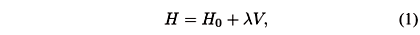where H0 denotes the free Hamiltonian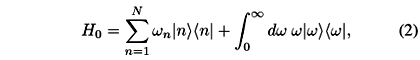and lV being the interaction Hamiltonianwith the coupling constant l. The eigenvalues wn of H0 were supposed not to be degenerate, i.e., wn < wn' for n < n'. Both |nñ and |wñ are the bound and scattering eigenstates of H0, respectively, and satisfy the orthonormality condition: án|n'ñ = dnn', áw| w 'ñ = d(w  w'), and án| wñ = 0 , where dnn' is Kronecker's delta and d(w  w') is Dirac's delta function. They also compose the completely orthonormal system (CONS) with the resolution of identity. In Eq. (3), vn (w) denotes the form factor characterizing the transition between |nñ and |wñ . In the latter discussion, we analyze the model with the assumption that the form factor vn (w) is analytic in a complex domain including the cut (0, ¥), square integrable, i.e.,and behaves likeas w ® 0 , where pn is a positive constant while qn is an appropriate one. The conditions (4) and (5) ensure that the integrald wvn (w)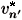(w)/w is definite. The large-energy condition (4) ensures thatd wvn (w)(w)/(z  w) is definite for all complex numbers z Ï [0, ¥). Both of the conditions are satisfied by several systems involving the spontaneous emission process of photons [8,9] and the photodetachment process of electrons [9-12]. Note that this small-energy condition (5) excludes the photoionization processes associated with the Coulomb interaction ; however, the formulation developed below could be applied to those cases.

The initial unstable-state |yñ of our interest is an arbitrary superposition of the discrete states |nñ,where cn's are complex numbers satisfying the normalization condition|cn |2 = 1. Then, the survival probability S(t) of the initial state |yñ , that is, the probability of finding the initial state in the state at a later time t, is defined by S(t) = |A(t)|2 . The A(t) denotes the survival amplitude of |yñ , i.e., A(t) = áy|eitH| yñ. The total probability P(t) that the state at the later time t remains in the subspace spanned by |nñ is also defined by P(t) = áy|eitH(|nñ án|)eitH|yñ . It is worth noting that P(t) > S(t) strictly holds for all times t, because the projection|nñ án| can be decomposed into the two parts of the projections |yñ áy| and P^ so that they are nonnegative and satisfy |y ñ áy|P^ = P^|yñ á y| = 0. One actually obtains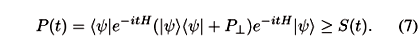In particular, in the SLA, i.e., N = 1, P(t) = S(t). The Hamiltonian (1) in general has the possibility of possessing not only the scattering eigenstates |ñ, but also the bound eigenstates [12,15,22-24]. However, the emitted particles detected in the decaying process are only brought from the initial component associated with the scattering eigenstates. We shall here confine ourselves to studying the decaying parts of A(t) and P(t), denoted by the same symbols asand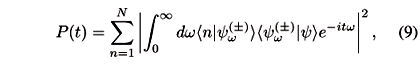respectively.

In order to estimate the long-time behaviors of both A(t) and P(t), let us evaluate the scattering eigenstates |ñ by solving the Lippmann-Schwinger equation,We here mention this procedure somewhat in detail to make an explanation in the self-consistent way. We first use the CONS to represent |ñ in the following form,where the coefficients(w) and f(±)(w' , w) are given by(w) = án|ñ and f(±)(w' , w) = áw'|ñ, respectively. Then, the scattering component á|yñ that appears in Eqs. (8) and (9) turns out to be represented simply byActing áw| from the left to Eqs. (10) and (11) and eliminating áw|ñ from both equations, one obtains,By the same way, acting án| from the left to Eqs. (10) and (11), and eliminating án|ñ from both equations again, at this time, one has,Then, substitution of Eq. (13) into (11) leads toTo determine(w), we can eliminate f(±)(w' , w) from Eqs. (13) and (14), and then have an algebraic equation for(w),wherewhich is the (n, n')-th component of the N × N matrix G1 (z), and snn' (z) is defined by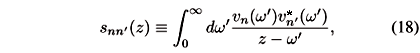for all z = reij (r > 0, 0 < j < 2p).

3 Small-energy behavior of the resolvent

Under the small-energy condition of Eq. (5), snn' (z) is guaranteed to be analytic in the whole complex plane except the cut [0, ¥). For the later convenience, G1(z) is defined as an inverse of G(z), where G(z) is assumed to be regular. Note that G(z) is nothing more than the reduced (or partial) resolvent Gnn'(z) = án|(H  z)1|n'ñ. One can confirm this fact by following the discussion in section 3.2 of Ref. . Since the behavior of A(t) and P(t) at long times are characterized by that of(w) at small energies, detrmined by Eq. (16), we need to estimate the small-energy behavior of G(z). Note that under the condition (5) we haveas w ® +0, where snn' (w±i0) = Inn' (w)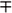ipvn (w)(w) and Inn' (w) º Pdw', where P denotes the principle value of the integral. The existence of Inn' (0) may be just guaranteed by the small-energy condition of Eq. (5) . Supposing that Gnn' is of the formas w ® +0, one obtains that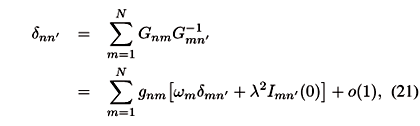We solve this equation by assuming that gnn' can be expanded for small l asBy substituting Eq. (23) into (22), it follows that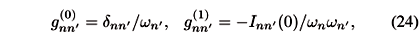and for j > 1where we have assumed that all wn does not vanish. Note that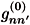andderived here accord with at least those for solvable cases, where G(z) is explicitly obtained [15,16]. We can then obtain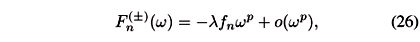withwherewhere p = min{pn}. With use of then instead of qn, we extracted only the dominant part of(w) at small w.

4 Long-time behavior of the survival and total probabilities

In this section, we shall examine the long-time behavior of both S(t) and P(t) with the various initial-states. The formula for S(t) (or A(t)) was obtained by Ref.  as follows,as t ® ¥, where i2p+1 = ei (2p+1)p/2, and G(z+1) =dxxz ex. Eq. (29) is derived by inserting Eqs. (12) and (26) into Eq. (8) by using the asymptotic method for the Fourier integral . One can clearly perceive A(t) ~ t2p1 , the power-decay law.

Let us first consider the higher-level effects on the long-time behavior of A(t) that starts from the localized initial state at the lowest level. For such an initial state, i.e., cn = dn1, Eq. (29) becomes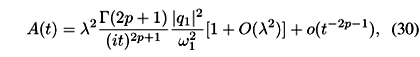where we supposed that

1¹ 0. Since there are no factors related to the higher levels in Eq. (30), it implies that the long-time asymptotic behavior of A(t) could agree with that in the SLA for a sufficiently small l. We can also find a special superposition of discrete states |nñ that maximizes the asymptotic form of A(t) at long times . It is worth noting that its dependence on the initial states only appears in Eq. (29) through the factorcn, which can be rewritten by an inner product asWe have here introduced an auxiliary vector defined byfrom which Eq. (29) readsas t ® ¥. With resort to the Schwarz inequality, we see that the maximum of the factor (31) is just attained by if and only if | yñ µ|cñ, i.e.,where c is an arbitrary complex number with |c| = 1. Therefore, preparing the initial state |yñ according to the above weights (34), we can maximize the asymptotic form of A(t) at long times. Substituting Eq. (34) into Eq. (33), one obtains thatOn the other hand, there are another kind of initial states that are coherently superposed to eliminate the factor (31) . This is indeed realized by the initial states orthogonal to |cñ, i.e.,In this case, the first term in the right-hand side (rhs) of Eq. (33) becomes zero. This fact may imply that A(t) for such an orthogonal state asymptotically decays faster than t2p1.

As same as for S(t), we can derive the asymptotic form of P(t) at long times, which is determined by the small-energy behavior of the integrand. By substituting Eqs. (12) and (26) into Eq. (9), and by applying the asymptotic method for the Fourier integral again, P(t) reads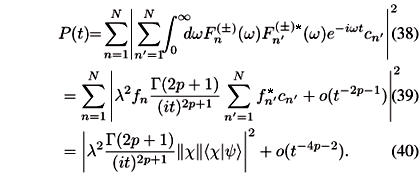It follows from Eqs. (33) and (40) that P(t)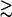S(t) holds at long times too, in the sense of the comparison between the first terms of the asymptotic expansion of P(t) and S(t).

We next examine the initial-state dependence of the long-time behavior of P(t). Let us first consider the higher-level effects on P(t) with the initial state localized at the lowest level. In this case, Eq. (40) reads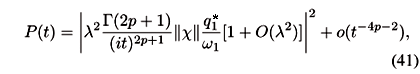with assumption that

1¹ 0. One clearly sees in Eq. (41) that the higher-level effect still remains through the factor ||c||, unlike the case for A(t). We note that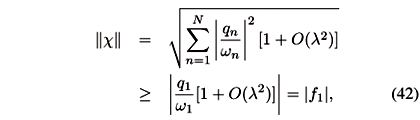which implies that as the number of levels N increases, the SLA with N = 1 may result in an underestimation of P(t) at long times. However, the resultant gain with a large N strictly depends on the system under the consideration: for instance, in the spontaneous emission process of photon from the hydrogen atom, ||c ||2/|f1|2~ 1.29 was obtained even for N = 50 . Furthermore, Eq. (40) tells us that the initial state maximizing the asymptotic form of P(t) at long times is the same as it for S(t), i.e., |cñ/|| c||. However, for such an initial state, we merely obtain that P(t) ~ S(t) at long times. One also finds from Eq. (40) such special initial-states that eliminate the first term of the rhs of the asymptotic expansion (40). This is in fact realized by the initial states orthogonal to |cñ as same as for the S(t). Therefore, P(t) for such an initial state could be expected to decay asymptotically faster than t4p2 too.

Befor concluding this section, we point out that the initial state extended over discrete states |nñ has the possibility of increasing the intensity of A(t) (P(t)) more than a localized one would. This possibility may be recognized as follows. The coupling lV makes a transition of the initial state, that is composed of the discrete states |nñ, into the continuum state |wñ, whereas the hermiticity of the Hamiltonian also allows an inverse process of making the state at a later time t get back into the subspace spanned by |nñ. In the latter process, there are various candidates for the reviving discrete-state. Repopulation of each discrete state can make the intensity of A(t) (P(t)) grow, provided that the initial state is composed of those discrete states. However, if the initial state only consists of a specific discrete state, the other discrete states composing the state at a later time t are thrown away without any contribution to A(t) (P(t)) . This is the reason why the decay of the A(t) (P(t)) for extended states can be relieved more than that for localized states.

5 Application to the excited states of the hydrogen atom

In order to illustrate our analysis developed in the foregoing sections, we consider the spontaneous emission process for the hydrogen atom interacting with the EM field [8,9]. In this case, we suppose that |nñ = |(n+1)pñÄ|0ñ, where |(n+1)pñ and |0ñ denote the (n+1)p-state of the atom and the vacuum state of the field respectively, and also |wñ = |1sñ Ä|1wñ, where |1sñ and |1wñ denote the 1s-state of the atom and the one-photon state respectively. Thus, an initially excited atom makes a transition to the ground state with the emission of a photon. We here choose only three excited levels: the 2p state, 3p state, and 4p state. Then, the form factors corresponding to the 2p1s, 3p1s, and 4p1s transitions become as follows [28,29],where L1 = 8.498 ×1018s1, L2 = (8/9)L1s1, and L3 = (10/12)L1s1 are the cut-off constants. Note that these form factors have different forms, however they behave in the same way at small energy like w1/2. The coupling constant is also given by l2 = 6.435 ×109. The embedded eigenvalues of H0 are defined by wn =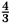W[1  (n+1)2] with W = 1.55 ×1016s1. To derive these form-factors, we represented |1wñ in the energy-angular momentum basis for photon  as in Ref. , incorporating the conservation of angular momentum and parity in each transition . As was emphasized in Ref. , these form-factors are surely analytic results without any approximation. The Hamiltonian (1) is then derived within the four-level approximation and the rotating-wave approximation.

In the following, we shall compare the long-time asymptotic-form of A(t) predicted by Eq. (33) and that of Acut(t), which we evaluate numerically. Acut(t) is defined byThe contour C runs clockwise around the half line {re7pi/4|0 < r < ¥} in the complex energy plane. This contour lies on the first Riemann sheet when it goes below the half line, and gets into the second Riemann sheet when it above the half line. Acut(t) is related to A(t) through the equation,where zp is in general the complex pole of áy|(H  z)1|yñ located in the region between the half lines [0, ¥) and {repi/4|0 < r < ¥} in the second Riemann sheet. In the weak-coupling case considered here, each of zp is in the neighborhood of wn, and thus the asymptotic form of Acut(t) and that of A(t) are expected to exhibit the same behavior at long times, where the power decay dominates over the exponential decay .

Let us first restrict ourselves to the two initial states: the localized state at the 2p level |1ñ and the maximizing state |cñ/||c ||. Figure 1 shows the time evolution of |Acut(t)|2 and the asymptote of |A(t)|2 for these initial-states. It is clearly seen that |Acut(t)|2's for these initial states approach to the corresponding asymptotes of |A(t)|2 parallel to t4, however the difference between them is very small . At t = 105, we obtain |Acut(t)/Aasp(t)|2~ 0.999 for these initial states. Is is worth stressing that this time is very earlier than 1/g1~ 1.36 ×1010the lifetime of the 2p state , where g1 = 2pl2|v1(w1)|2 + O(l4) ~ 6.268 ×108s1 .

We next choose the two special states, |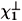ñ and |ñ, as the initial state |yñ . The former is defined byand the latter isso that they eliminate the factor (31) and satisfy the relations that ác|ñ = 0, ác|ñ = 0 , and á|ñ = 0 . Figure 2 shows that the time evolution of |Acut(t)|2 for these initial states and for the maximizing initial state. We clearly find that, as was seen in Fig. 1, |Acut(t)|2 for the maximizing initial state (solid curve) asymptotically decays like t4, whereas |Acut(t)|2 for other initial state (long-dashed and short-dashed curves) follow another decay-law faster than t4. They seem to be fitted with the power law t8. For the comparison, we also depict in Fig. 2 the two straight lines 150.0 ×(L1t)8 and 30.0 ×(L1t)8 (solid lines), to which |Acut(t)|2 for the initial state |ñ and |ñ approach respectively in this time region.

6 Concluding remarks

We have considered the long-time behavior of the unstable multilevel systems and examined the asymptotic behavior of not only the survival S(t) but also the total P(t) probabilities for an arbitrary initial state in the long-time region, where both S(t) and P(t) obey a power decay law. For the initial state localized at the lowest level, we have found that the SLA results in an underestimation of the asymptotic form of P(t) unlike the result for S(t). We have also discovered two kinds of special initial-state. One of them maximizes the asymptotic form of P(t) at long times, and actually the same state that maximizes S(t). However, for such an initial state, we only obtain that P(t) ~ S(t) at long times, even though P(t) > S(t) holds for all times t. The other initial state eliminates the first term of the asymptotic expansion of P(t), and also plays the same role for S(t). In addition, we numerically confirm the previous results for S(t)  in consideration of the spontaneous emission process for the hydrogen atom. Then, we show not only the t4-decay of S(t) but also a more faster decay, the latter of which is naively fitted by a power-decay law t8. However, we still do not accomplish the derivation of the asymptotic forms for such faster decays. To this end, it will be needed to take into account the zero-energy resonance and the zero-energy eigenstate with an appropriate state space [34,35,36]. We hope to deal with this issue in the future.

7 Acknowledgments

The author would like to express his gratitude to the organizers of the Second International Workshop DICE2004 Castello di Piombino (Tuscany), September 1-4, 2004, From Decoherence and Emergent Classicality to Emergent Quantum Mechanics. He also would like to thank Professor I. Ohba and Professor H. Nakazato for useful and helpful discussions. This work is partly supported by a Grant for The 21st Century COE Program at Waseda University from the Ministry of Education, Culture, Sports, Science and Technology, Japan.

References

 G. Gamow, Z. Phys. 51, 204 (1928).

 For a review, see, for example, H. Nakazato, M. Namiki, and S. Pascazio, Int. J. Mod. Phys. B 10, 247 (1996).

 L. A. Khalfin, Zh. Eksp. Theor. Fiz. 33, 1371 (1957) [ Sov. Phys. JETP 6, 1053 (1958)].

 S. R. Wilkinson, et al, Nature (London) 387, 575 (1997).

 P. T. Greenland, Nature (London) 335, 298 (1988).

 K. O. Friedrichs, Commun. Pure Appl. Math. 1, 361 (1948).

 P. Exner, Open Quantum Systems and Feynman Integrals (Reidel, Doredrecht, 1985).

 P. Facchi and S. Pascazio, Phys. Lett. A 241, 139 (1998).

 I. Antoniou, E. Karpov, G. Pronko, and E. Yarevsky, Phys. Rev. A 63, 062110 (2001).

 K. Rza\. zewski, M. Lewenstein, and J. H. Eberly, J. Phys. B 15, L661 (1982).

 S. L. Haan and J. Cooper, J. Phys. B 17, 3481 (1984).

 H. Nakazato, in ''Fundamental Aspects of Quantum Physics'', edited by L. Accardi and S. Tasaki (World Scientific, New Jersey, 2003).

 E. Frishman and M. Shapiro, Phys. Rev. Lett. 87, 253001 (2001); Phys. Rev. A 68, 032717 (2003).

 I. Antoniou, E. Karpov, G. Pronko, and E. Yarevsky, Int. J. Theor. Phys. 42, 2403 (2003).

 I. Antoniou, E. Karpov, G. Pronko, and E. Yarevsky, quant-ph/0402210 (2004).

 E. B. Davies, J. Math. Phys. 15, 2036 (1974).

 M. Miyamoto, Phys. Rev. A 70, 032108 (2004).

 K. Unnikrishnan, Am. J. Phys. 65, 526 (1997); 66, 632 (1998); F. Lillo and R. N. Mantegna, Phys. Rev. Lett. 84, 1061 (2000); 84, 4516 (2000); J. A. Damborenea, I. L. Egusquiza, and J. G. Muga, Am. J. Phys. 70, 738 (2002); M. Miyamoto, J. Phys. A 35, 7159 (2002); Phys. Rev. A 68, 022702 (2003).

 M. Miyamoto, Phys. Rev. A 69, 042704 (2004).

 F. Remacle and R. D. Levine, J. Chem. Phys. 104, 1399 (1996).

 E. P. Wigner, Phys. Rev. 73, 1002 (1948).

 L. P. Horwitz and J.-P. Marchand, Rocky Mountain J. Math. 1, 225 (1971).

 A. G. Kofman G. Kurizki and B. Sherman, J Mod Opt. 41, 353 (1994).

 For the multilevel cases, if all eigenvalues wn of H0 are positive and non-degenerate, and the coupling constant l is sufficiently weak, then we can show that no negative eigenvalues of H exist.

 In fact, by using the technique in the proof of Proposition 3.2.2. in Ref. , we can show under our assumption to $v_n(\omega)$ that \$-\int^{\infty}_0 d\omega^\prime v_n(\omega^\prime)v^*_{n^\prime}(\omega^\prime) / \omega^\prime = \lim_{omega |uprrow 0} S_{nn^\prime}(\omega)= \lim_{\omega \downarrow 0} I_{nn^\prime}(\omega).

 E. T. Copson, Asymptotic Expansions (Cambridge Univ. Press, Cambridge, 1965), Chap. 3.

 This interpretation is not necessarily applied to all of the time region. The reason is that such repopulation processes are naively expected to occur under the dominance of the terms of O(l2) for our interaction Hamiltonian lV. This does not contradict the behavior of A(t) (and P(t)) at long times .

 H. E. Mose, Phys. Rev. A 8, 1710 (1973).

 J. Seke, Physica A 203, 269 (1994).

 A. I. Akhiezer and V. B. Berestetskii, Quantum Electrodynamics (Interscience, New York, 1965).

 Note that the form factors obtained by Ref.  are described by the one-photon state with a definite helicity. Thus, to use those results, we need to rewrite such a state in the parity representation . However, this change makes a difference only in the pre-factor of the results of .

 The transition time tep from the exponential to the power decay law is, e.g., approximately 100 lifetimes for the localized state at the 2p level [8,9]. At this time, we obtain, |A(tep)|2~ 4.41 ×1055, an extremely small value .

 P. Facchi, Ph. D. thesis, Universit\' a degli Studi di Bari, 2000.

 J. Rauch, Comm. Math. Phys. 61, 149 (1978).

 A. Jensen and T. Kato, Duke Math. J. 46, 583 (1979).

 M. Murata, J. Funct. Anal. 49, 10 (1982).

Received on 8 January, 2005

•  G. Gamow, Z. Phys. 51, 204 (1928).
•  For a review, see, for example, H. Nakazato, M. Namiki, and S. Pascazio, Int. J. Mod. Phys. B 10, 247 (1996).
•  L. A. Khalfin, Zh. Eksp. Theor. Fiz. 33, 1371 (1957) [
• Sov. Phys. JETP 6, 1053 (1958)].
•  S. R. Wilkinson, et al, Nature (London) 387, 575 (1997).
•  P. T. Greenland, Nature (London) 335, 298 (1988).
•  K. O. Friedrichs, Commun. Pure Appl. Math. 1, 361 (1948).
•  P. Exner, Open Quantum Systems and Feynman Integrals (Reidel, Doredrecht, 1985).
•  P. Facchi and S. Pascazio, Phys. Lett. A 241, 139 (1998).
•  I. Antoniou, E. Karpov, G. Pronko, and E. Yarevsky, Phys. Rev. A 63, 062110 (2001).
•  K. Rza\. zewski, M. Lewenstein, and J. H. Eberly, J. Phys. B 15, L661 (1982).
•  S. L. Haan and J. Cooper, J. Phys. B 17, 3481 (1984).
•  H. Nakazato, in ''Fundamental Aspects of Quantum Physics'', edited by L. Accardi and S. Tasaki (World Scientific, New Jersey, 2003).
•  E. Frishman and M. Shapiro, Phys. Rev. Lett. 87, 253001 (2001);
• Phys. Rev. A 68, 032717 (2003).
•  I. Antoniou, E. Karpov, G. Pronko, and E. Yarevsky, Int. J. Theor. Phys. 42, 2403 (2003).
•  E. B. Davies, J. Math. Phys. 15, 2036 (1974).
•  M. Miyamoto, Phys. Rev. A 70, 032108 (2004).
•  K. Unnikrishnan, Am. J. Phys. 65, 526 (1997);
• 66, 632 (1998); F. Lillo and R. N. Mantegna, Phys. Rev. Lett. 84, 1061 (2000);
• 84, 4516 (2000); J. A. Damborenea, I. L. Egusquiza, and J. G. Muga, Am. J. Phys. 70, 738 (2002);
• M. Miyamoto, J. Phys. A 35, 7159 (2002);
• Phys. Rev. A 68, 022702 (2003).
•  M. Miyamoto, Phys. Rev. A 69, 042704 (2004).
•  F. Remacle and R. D. Levine, J. Chem. Phys. 104, 1399 (1996).
•  E. P. Wigner, Phys. Rev. 73, 1002 (1948).
•  L. P. Horwitz and J.-P. Marchand, Rocky Mountain J. Math. 1, 225 (1971).
•  A. G. Kofman G. Kurizki and B. Sherman, J Mod Opt. 41, 353 (1994).
•  E. T. Copson, Asymptotic Expansions (Cambridge Univ. Press, Cambridge, 1965), Chap. 3.
•  H. E. Mose, Phys. Rev. A 8, 1710 (1973).
•  J. Seke, Physica A 203, 269 (1994).
•  A. I. Akhiezer and V. B. Berestetskii, Quantum Electrodynamics (Interscience, New York, 1965).
•  P. Facchi, Ph. D. thesis, Universit\' a degli Studi di Bari, 2000.
•  J. Rauch, Comm. Math. Phys. 61, 149 (1978).
•  A. Jensen and T. Kato, Duke Math. J. 46, 583 (1979).
•  M. Murata, J. Funct. Anal. 49, 10 (1982).

# Publication Dates

• Publication in this collection
06 Sept 2005
• Date of issue
June 2005